DHMM 是 Discrete Hidden Markov Model 的簡稱，對語音辨識的應用而言，我們的目標，就是事先使用大量語料，對每一個辨識語句建立一個 DHMM，然後當我們取得使用者輸入的測試語句後，即對測試語句進行端點偵測及 MFCC 特徵擷取，然後再將 MFCC 送至各個辨識語句所形成的 DHMM，算出每一個模型的機率值，機率值最大的 DHMM，所對應的辨識語句就是此系統的辨識結果。

1. 收集語料：由於我們的目標是語者無關的語音辨識，所以我們所收集的語料要盡量涵蓋各種可能的變化，例如：
• 錄音的人：各種不同的人，包含男女老少、高矮胖瘦等，以及各種南腔北調。
• 錄音裝置：取用不同的裝置，例如不同的麥克風、不同的音效卡等。
• 周遭環境：除了要在安靜的辦公室進行錄音外，也可以在吵雜的環境錄音，以增強語音辨識系統對於雜訊的抵抗力。
2. 辨識系統的設計和測試：這也是我們下面的重點。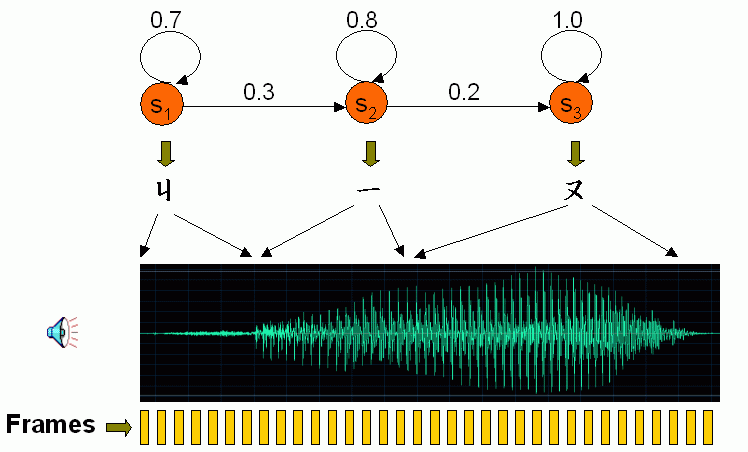• dim：每一個音框所對應的語音特徵向量的維度（例如基本的 MFCC 有 12 維）。
• stateNum：一個 DHMM 的狀態個數
• symbolNum：符號的個數，也就是 VQ 後的群數

HMM 的參數，就是指「狀態機率」和「轉移機率」，說明如下：

• 我們通常以矩陣 A 來表示轉移機率，其維度是 stateNum x stateNum，其中 A(i, j) 即是指由狀態 i 跳到狀態 j 的機率值。例如在上圖中，由狀態 1 跳到狀態 2 的機率是 0.3，因此 A(1, 2) = 0.3。一般而言，A(i, j) 滿足下列條件：
• 在進行次轉移時，我們只允許搜尋路徑跳到下一個相鄰的狀態，因此 A(i, j) = 0 if j≠i and j≠i+1。
• 對於某一個狀態而言，所有的轉移機率總和為 1，因此 A(i, i) + A(i, i+1) = 1。
• 我們通常以矩陣 B 來表示狀態機率，其維度是 symbolNum x stateNum，B(k,j) 即是指符號 k 隸屬於狀態 j 的機率值。換句話說，B 定義了由「符號」到「狀態」的機率，因此給定第 i 個音框，我們必須先由 O(i) 找出這個音框所對應的符號 k，然後再由 B(k,j) 找出此音框屬於狀態 j 的機率。

1. 目標函數：定義 D(i, j) 是 t(1:i) 和 r(1:j) 之間的最大的機率，t(1:i) 是前 i 個音框的特徵向量所成的矩陣，r(1:j) 則是由前 j 個狀態所形成的 DHMM，對應的最佳路徑是由 (1, 1) 走到 (i, j)。
2. 遞迴關係：D(i, j) = B(O(i), j) + max{D(i-1, j)+A(j, j), D(i-1, j-1)+A(j-1, j)}
3. 端點條件：D(1, j) = p(1, j) + B(O(1), j), j = 1 ~ stateNum
4. 最後答案：D(m, n)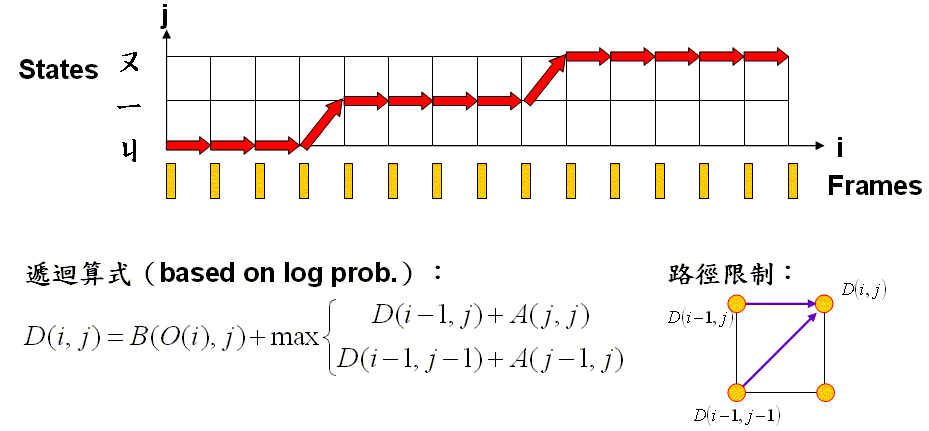• 以加法來取代乘法，降低計算的複雜度。
• 避開了由於連乘所造成的數值誤差。

• 在 DHMM，若要計算 B(O(i),j)，我們必須先找到音框 i 所對應的符號 O(i)，然後在經由查表法查到此符號 O(i) 屬於狀態 j 的機率是 B(O(i), j)。
• 在 CHMM，B(O(i),j) 是由一個連續的機率密度函數所定義，請見下一小節說明。

1. 將所有的訓練語句切出音框，並將每一個音框轉成語音特徵向量，例如 39 維的 MFCC。
2. 對所有語音特徵向量進行VQ，找出每一個向量所對應的 symbol（此即為對應的中心點或codeword）
3. 先猜一組 A 和 B 的啟始值。如果沒有人工的標示資料，我們可以使用簡單的「均分法」，示意圖如下：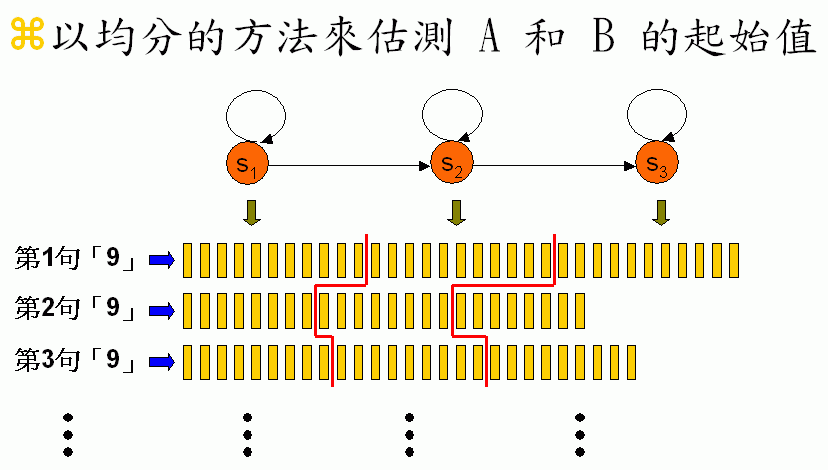4. 反覆進行下列兩步驟，直到收斂
• Viterbi decoding：在 A 和 B 固定的情況下，利用 Viterbi decoding，找出 n 句「九」的語料所對應的 n 條最佳路徑。
• Re-estimation：利用這 n 條最佳路徑，重新估算 A 和 B。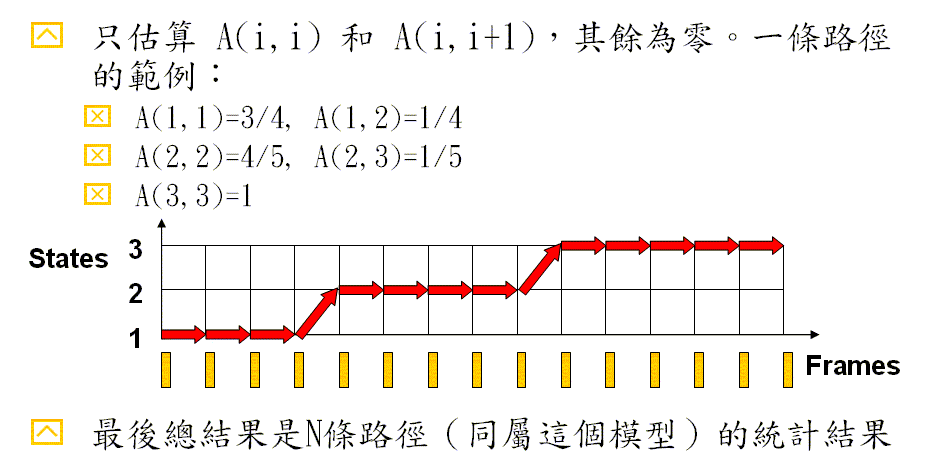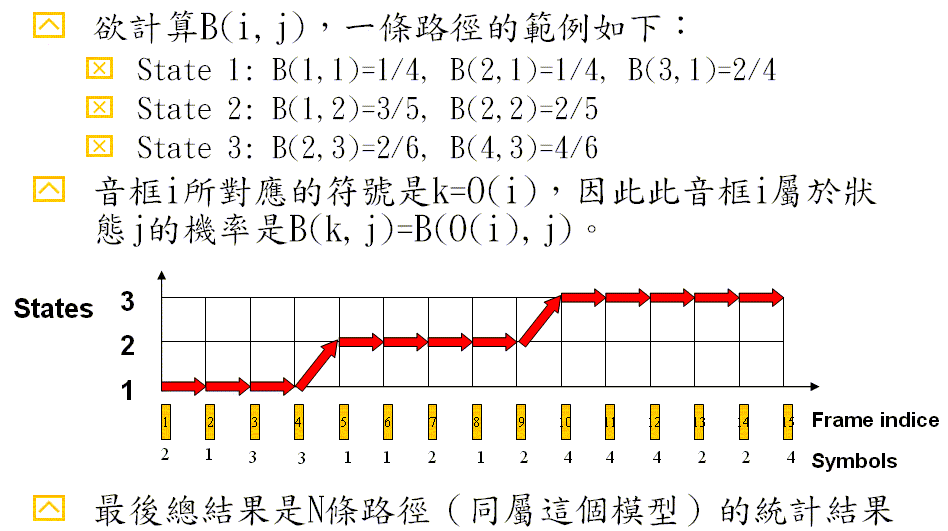• State 1: D(1,1)=B(2,1)=1/4, D(2,1)=B(1,1)=1/4, D(3,1)=B(3,1)=2/4, D(4,1)=B(3,1)=2/4
• State 2: D(5,2)=B(1,1)=3/5, D(6,2)=B(1,1)=3/5, D(7,2)=B(2,1)=2/5, D(8,2)=B(1,1)=3/5, D(9,2)=B(2,1)=2/5
• State 2: D(10,3)=B(4,3)=4/6, D(11,3)=B(4,3)=4/6, D(12,3)=B(4,3)=4/6, D(13,3)=B(2,3)=2/6, D(14,3)=B(2,3)=2/6, D(15,3)=B(4,3)=4/6

1. 在 A, B 固定時，Viterbi decoding 會找出最佳的路徑，使 P(A, B, Path) 有最大值
2. 在路徑（Path）固定時，Re-estimation 會找出最佳的 A, B 值以使 P(A, B, Path)有最大值

• State 1: D(1,1)A(1,1)D(2,1)A(1,1)D(3,1)A(1,1)D(4,1)A(1,2) = A(1,1)3A(1,2)D(1,1)D(2,1)D(3,1)D(4,1)
• State 2: D(5,2)A(2,2)D(6,2)A(2,2)D(7,2)A(2,2)D(8,2)A(2,2)D(9,2)A(2,3) = A(2,2)4A(2,3)D(5,2)D(6,2)D(7,2)D(8,2)D(9,2)
• State 3: D(10,3)A(3,3)D(11,3)A(3,3)D(12,3)A(3,3)D(13,3)A(3,3)D(14,3)A(3,3)D(15,3)A(3,3) = A(3,3)6D(10,3)D(11,3)D(12,3)D(13,3)D(14,3)D(15,3)

• p: self-transition prob.（未知）
• q: next-transition prob.（未知）
• a: self-transition count（已知）
• b: next-transition count（已知）

Max J(p, q) = pa qb s.t. p≧0, q≧, and p + q = 1

x1 + x2 + ... + xn ≧ n (x1 x2 ... xn)1/n

p/a + p/a + ... + q/b + q/b ... ≧ (a + b) [(p/a)a(q/b)b]1/(a+b)
1 ≧ (a + b) [(p/a)a(q/b)b]1/(a+b)

• State prob: p, q, r（未知）
• Symbol count: a, b, c（已知）

J(p, q, r) = pa qb rc

Data Clustering and Pattern Recognition (資料分群與樣式辨認)